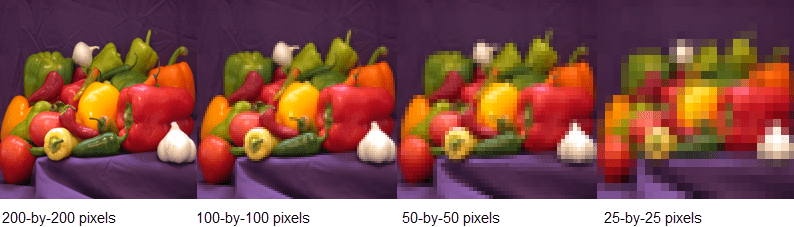# Image-to-Image Regression in Deep Network Designer

This example shows how to use Deep Network Designer to construct and train an image-to-image regression network for super resolution.

Spatial resolution is the number of pixels used to construct a digital image. An image with a high spatial resolution is composed of a greater number of pixels and as a result the image contains greater detail. Super resolution is the process of taking as input a low resolution image and upscaling it into a higher resolution image. When you work with image data, you might reduce the spatial resolution to decrease the size of the data, at the cost of losing information. To recover this lost information, you can train a deep learning network to predict the missing details of an image. In this example, you recover 28-by-28 pixel images from images that were compressed to 7-by-7 pixels.This example uses the digits data set, which consists of 10,000 synthetic grayscale images of handwritten digits. Each image is 28-by-28-by-1 pixels.

Load the data and create an image datastore.

```dataFolder = fullfile(toolboxdir('nnet'),'nndemos','nndatasets','DigitDataset'); imds = imageDatastore(dataFolder, ... 'IncludeSubfolders',true, .... 'LabelSource','foldernames');```

Use the `shuffle` function to shuffle the data prior to training.

`imds = shuffle(imds);`

Use the `splitEachLabel` function to divide the image datastore into three image datastores containing images for training, validation, and testing.

`[imdsTrain,imdsVal,imdsTest] = splitEachLabel(imds,0.7,0.15,0.15,'randomized');`

Normalize the data in each image to the range [0,1]. Normalization helps stabilize and speed up network training using gradient descent. If your data is poorly scaled, then the loss can become NaN and the network parameters can diverge during training.

```imdsTrain = transform(imdsTrain,@(x) rescale(x)); imdsVal = transform(imdsVal,@(x) rescale(x)); imdsTest = transform(imdsTest,@(x) rescale(x));```

### Generate Training Data

Create a training data set by generating pairs of images consisting of upsampled low resolution images and the corresponding high resolution images.

To train a network to perform image-to-image regression, the images need to be pairs consisting of an input and a response where both images are the same size. Generate the training data by downsampling each image to 7-by-7 pixels and then upsampling to 28-by-28 pixels. Using the pairs of transformed and original images, the network can learn how to map between the two different resolutions.

Generate the input data using the helper function `upsampLowRes`, which uses `imresize` to produce lower resolution images.

```imdsInputTrain = transform(imdsTrain,@upsampLowRes); imdsInputVal= transform(imdsVal,@upsampLowRes); imdsInputTest = transform(imdsTest,@upsampLowRes);```

Use the `combine` function to combine the low and high resolution images into a single datastore. The output of the `combine` function is a `CombinedDatastore` object.

```dsTrain = combine(imdsInputTrain,imdsTrain); dsVal = combine(imdsInputVal,imdsVal); dsTest = combine(imdsInputTest,imdsTest);```

### Create Network Architecture

Create the network architecture using the `unetLayers` function from Computer Vision Toolbox™. This function provides a network suitable for semantic segmentation that can be easily adapted for image-to-image regression.

Create a network with input size 28-by-28-by-1 pixels.

`layers = unetLayers([28,28,1],2,'encoderDepth',2);`

Edit the network for image-to-image regression using Deep Network Designer.

`deepNetworkDesigner(layers);`

In the Designer pane, replace the softmax and pixel classification layers with a regression layer from the Layer Library.Select the final convolutional layer and set the `NumFilters` property to `1`.The network is now ready for training.

### Import Data

Import the training and validation data into Deep Network Designer.

In the Data tab, click Import Data > Import Custom Data and select `dsTrain` as the training data and `dsVal` as the validation data. Import both datastores by clicking Import.Deep Network Designer displays the pairs of images in the combined datastore. The upscaled low resolution input images are on the left, and the original high resolution response images are on the right. The network learns how to map between the input and the response images.### Train Network

Select the training options and train the network.

In the Training tab, select Training Options. From the Solver list, select `adam`. Set MaxEpochs to `10`. Confirm the training options by clicking OK.Train the network on the combined datastore by clicking Train.As the network learns how to map between the two images the validation root mean squared error (RMSE) decreases.

Once training is complete, click Export to export the trained network to the workspace. The trained network is stored in the variable `trainedNetwork_1`.

### Test Network

Evaluate the performance of the network using the test data.

Using `predict`, you can test if the network can produce a high resolution image from a low resolution input image that was not included in the training set.

```ypred = predict(trainedNetwork_1,dsTest); for i = 1:8 I(1:2,i) = read(dsTest); I(3,i) = {ypred(:,:,:,i)}; end```

Compare the input, predicted, and response images.

```subplot(1,3,1) imshow(imtile(I(1,:),'GridSize',[8,1])) title('Input') subplot(1,3,2) imshow(imtile(I(3,:),'GridSize',[8,1])) title('Predict') subplot(1,3,3) imshow(imtile(I(2,:),'GridSize',[8,1])) title('Response')```The network successfully produces high resolution images from low resolution inputs.

The network in this example is very simple and highly tailored to the digits data set. For an example showing how to create a more complex image-to-image regression network for everyday images, see Increase Image Resolution Using Deep Learning.

### Supporting Functions

```function dataOut = upsampLowRes(dataIn) temp = dataIn; temp = imresize(temp,[7,7],'method','bilinear'); dataOut = {imresize(temp,[28,28],'method','bilinear')}; end```